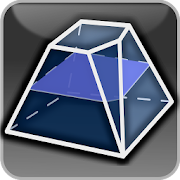# Geometryx: Geometry - CalculatorEveryone
5,266

Geometryx is an application that allows you to calculate quickly and easily the most important values and parameters of plane and solid figures and shapes.
The application calculates an area, perimeter, circumference, diagonal length, volume, coordinates of the geometric centroid, height, side lenght, angle (acute, right, obtuse, straight, reflex), radius (inner, outer), edges, arc length, line segments, base area, lateral surface area and total surface area of three-dimensional geometric shapes.
Geometryx is a simple calculator using trigonometric functions, Pythagorean theorem and Thales' theorem.

Geometryx also includes the most significant geometric formulas and equations that help you solve any problems and tasks in geometry.
Thanks to this application, the geometry will become very simple. Geometryx will be helpful for students, teachers, scientists, engineers, technicians, and anyone who has contact with geometry.
This geometric calculator uses sophisticated mathematical algorithms to solve various complex combinations of mathematical and geometric problems. Easy to use for everyone.

Geometryx = Great Geometry EXperience !

List of plane and solid figures contained in the application:

Planimetry ( 2D Geometry ):
• Square
• Rectangle
• Parallelogram
• Trapezoid
• Scalene triangle
• Isosceles triangle
• Equilateral triangle
• Right triangle
• Simple polygon
• Regular convex polygon
• Circle / Disk
• Annulus
• Annular sector
• Circular sector
• Circular segment
• Ellipse
• Ellipse segment
• Cubic function
• Intercept theorem
• Kite
• Angles and Trigonometry
• Rhombus
• Incircle and circumcircle of a triangle

• Stereometry ( 3D Geometry ):
• Cube
• Cuboid
• Right prism
• Oblique prism
• Right circular cylinder
• Oblique circular cylinder
• Cylindrical segment
• Cylindrical wedge
• Pyramid
• Frustum
• Obelisk
• Prismatoid
• Right circular cone
• Oblique circular cone
• Right truncated cone
• Oblique truncated cone
• Elliptic cone
• Truncated elliptic cone
• Sphere / Disk
• Spherical sector
• Sphere cap
• Spherical segment
• Ellipsoid
• Paraboloid of revolution
• Toroid
• Torus
• Right hollow cylinder
• Rectangular pipe
• Prism with a regular base
• Pyramid with a regular base
• Elliptical Cylinder
• Spherical Wedge
• Regular Tetrahedron
• Regular Octahedron
• Regular Dodecahedron
• Regular Icosahedron
• Wedge
• Barrel
Collapse

## Reviews

Review policy and info
4.5
5,266 total
5
4
3
2
1

## What's New

- added Hindi language (translation thanks to Jatin Kumar Varshney)
- app size optimisation
Collapse

Updated
October 31, 2020
Size
Varies with device
Installs
500,000+
Current Version
Varies with device
Requires Android
Varies with device
Content Rating
Everyone
Permissions
Offered By
famobix